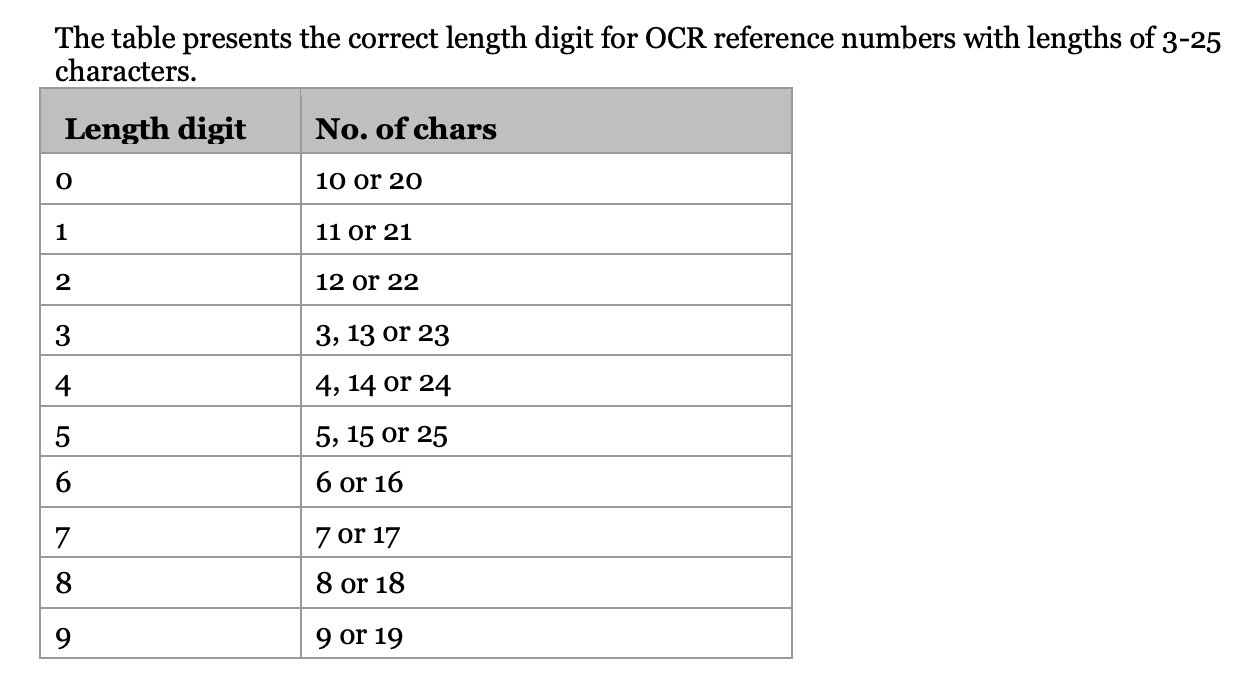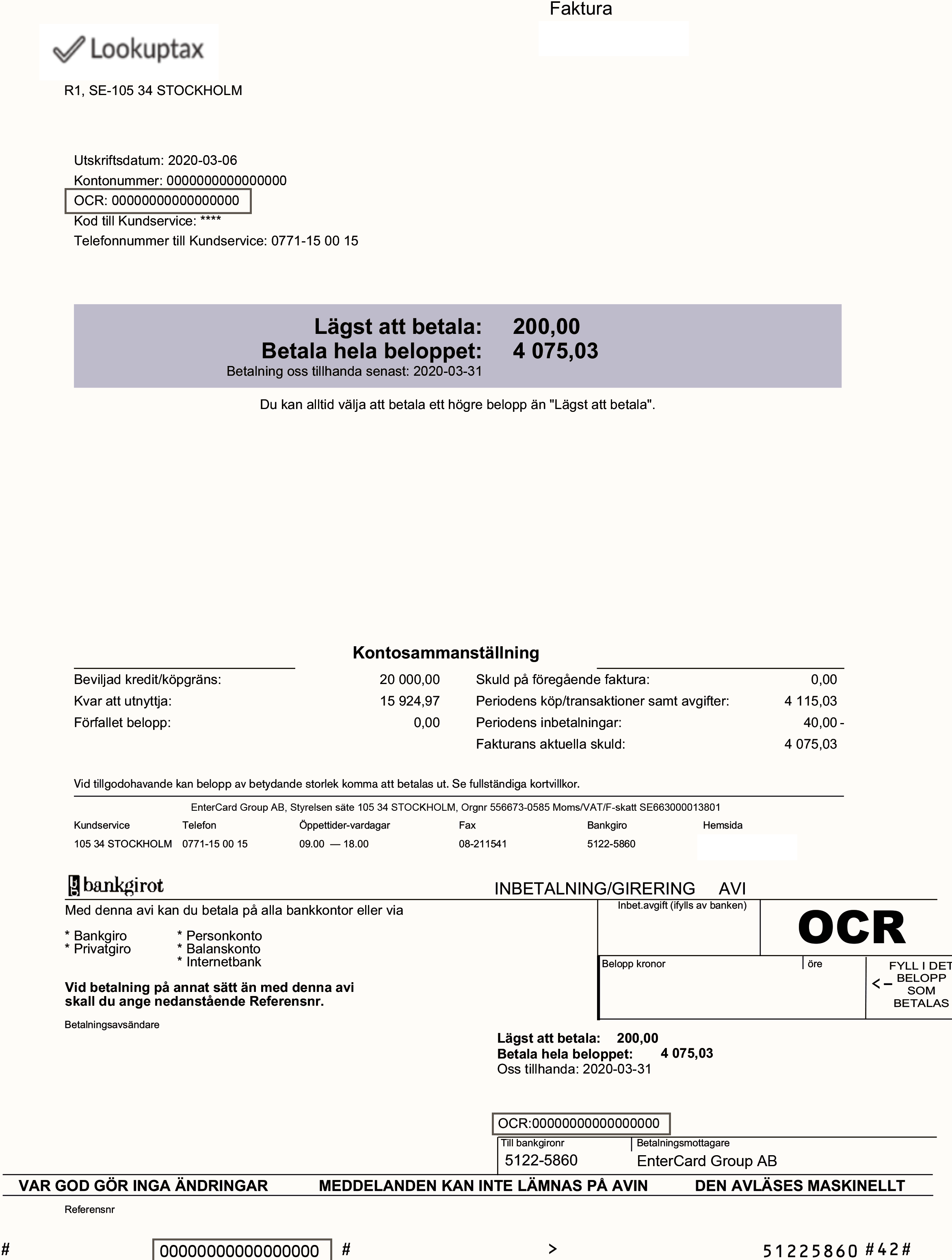# OCR number in Sweden explained

## What is the OCR number?​

The OCR number in Sweden is used to associate payments with the right customer and the right invoice. It serves the exact same purpose that the KID number serves in Norway.

## Format of the OCR number​

The format to be followed is Leading 0’s (if any) + Next Number in sequence + Check digit

Number of digits — 3 to 25 digits including the check digit. The last digit in OCR is the check digit. The OCR number must contain only digits ( use numbers from 0-9) and should not be alphanumeric. Merchant enters into an agreement with the bank to decide the number of digits in the reference number.

Rules to build the OCR number — OCR numbers can contain customer ID and/or invoice number to identify customer and invoice but this is not mandatory. It is however recommended to use these to make the reconciliation of payments with invoices easier.

Check digit calculation algorithm - Mod 10 algorithm. Use the Mod 10 calculator to validate the OCR number that you generate.

Next number in sequence - A random input number that will be used as the starting number to generate the reference number. ( This is obvious because how would you generate OCR if you don't have something to begin with. This can be the invoice number too if you don't want to use a new number. Just make sure that you are only using digits and the invoice number is not alphanumeric.)

Leading 0’s - If the final number of digits in the Variable part is less than the ‘Number of digits allowed in the reference number’ then we will add 0’s at the front to match.

OCR controls

There are 4 levels of checks to see if the OCR number is correct.See Section 5.1.2 here in this user manual by a European clearing house Bankgirot —

1. Soft control: If the payment contains an incorrect OCR reference number the payer receives an error message indicating that the OCR reference number has been entered incorrectly but that the payment can still be made. (Notice how there is no length control here). Basically this will accept any value including null value.

2. Hard Control: That the OCR reference number contains the correct Check digit.

3. Hard Control with variable length : It checks for the correct Check digit and also checks for the correct Length digit.

4. Hard control with fixed length: It checks for the correct Check digit and also checks for the Length of the reference number. This length is agreed upon with the bank and a maximum of 2 lengths can be chosen. Length digit is not necessary in this case because unlike Variable length, only 2 lengths are acceptable.

##### note
• Definition of length digit : Specifies the expected length of the OCR reference number. The length is specified in the second from last digit of the OCR reference number. The payment is not accepted by the Internet bank if the control returns an error. Example: If the reference number is 5, 15 or 25 digits in length, the second from last digit is 5.Calculating Length Digit

#### Let’s take some examples to see how the final generated number will look like​

Case 1: OCR controls without variable length

1. Total Number of digits in OCR - 10
2. Next number in OCR sequence - 567
3. Check digit algorithm - Mod 10

Final OCR number generated = 0000005678

Case 2: OCR controls with variable length

1. Total Number of digits in OCR - 10
2. Next number in OCR sequence - 567
3. Check digit algorithm - Mod 10
4. Length digit - 0

Final OCR number generated = 0000056705

## Sample Invoice with OCR number​Sample Invoice with OCR number in Sweden